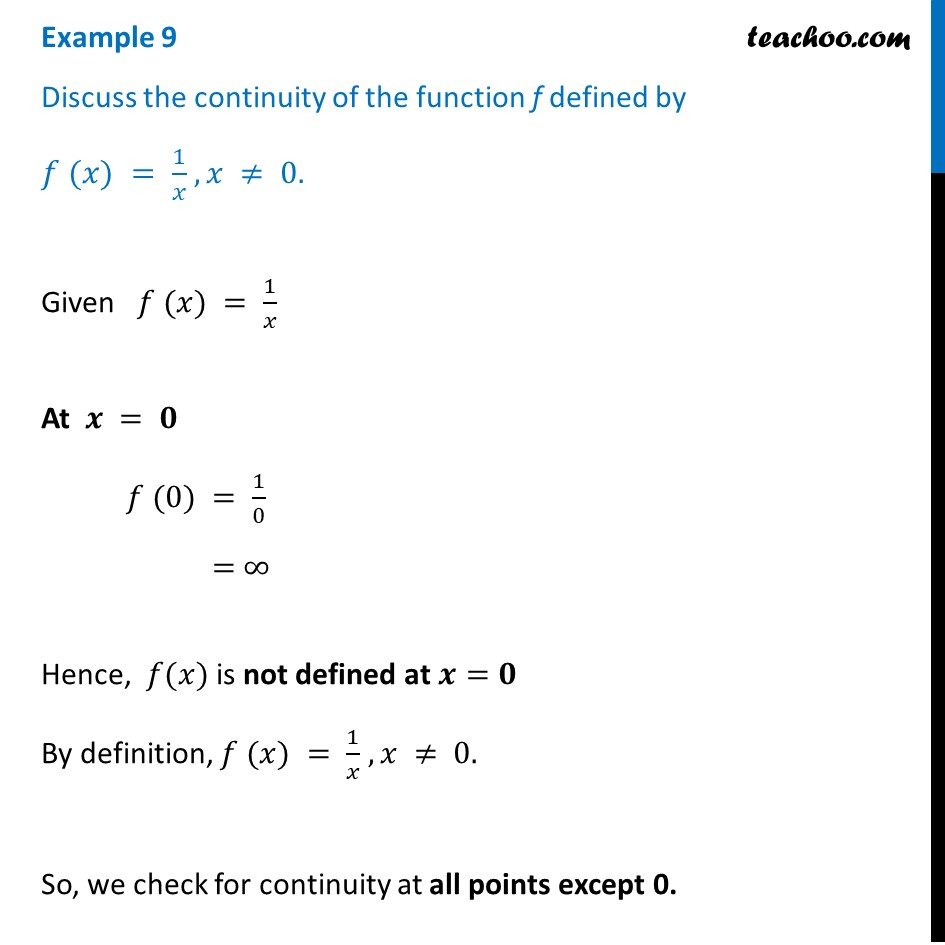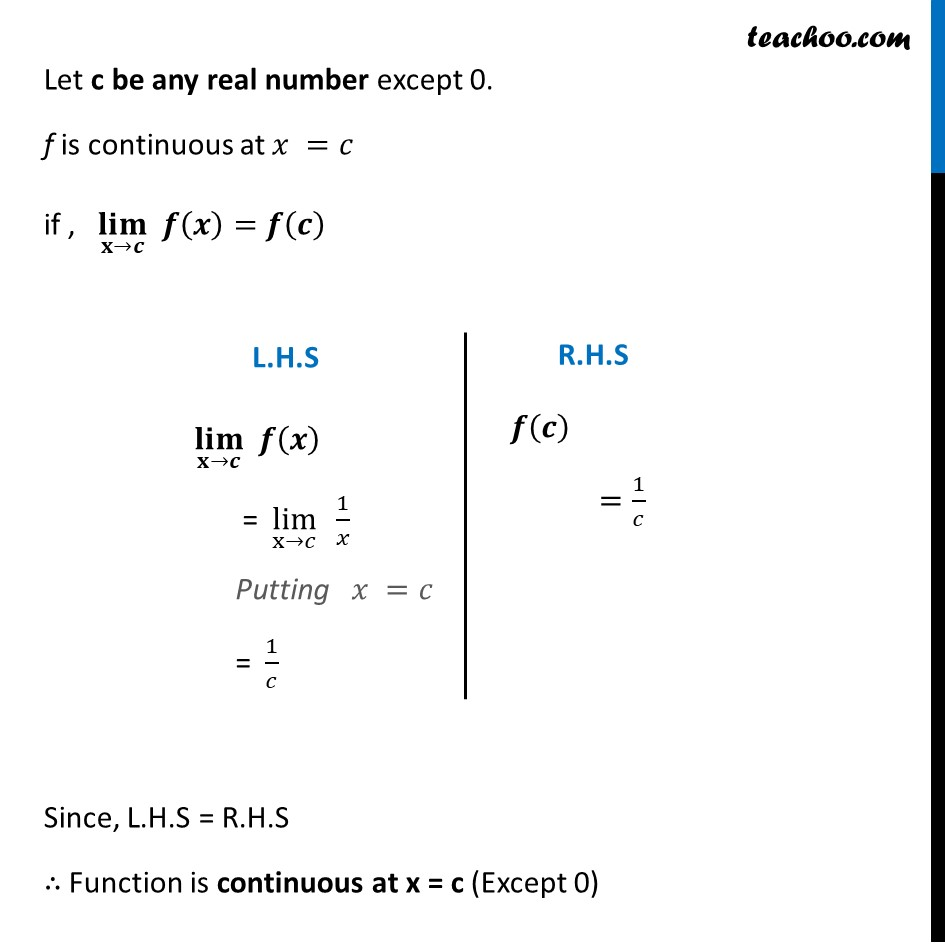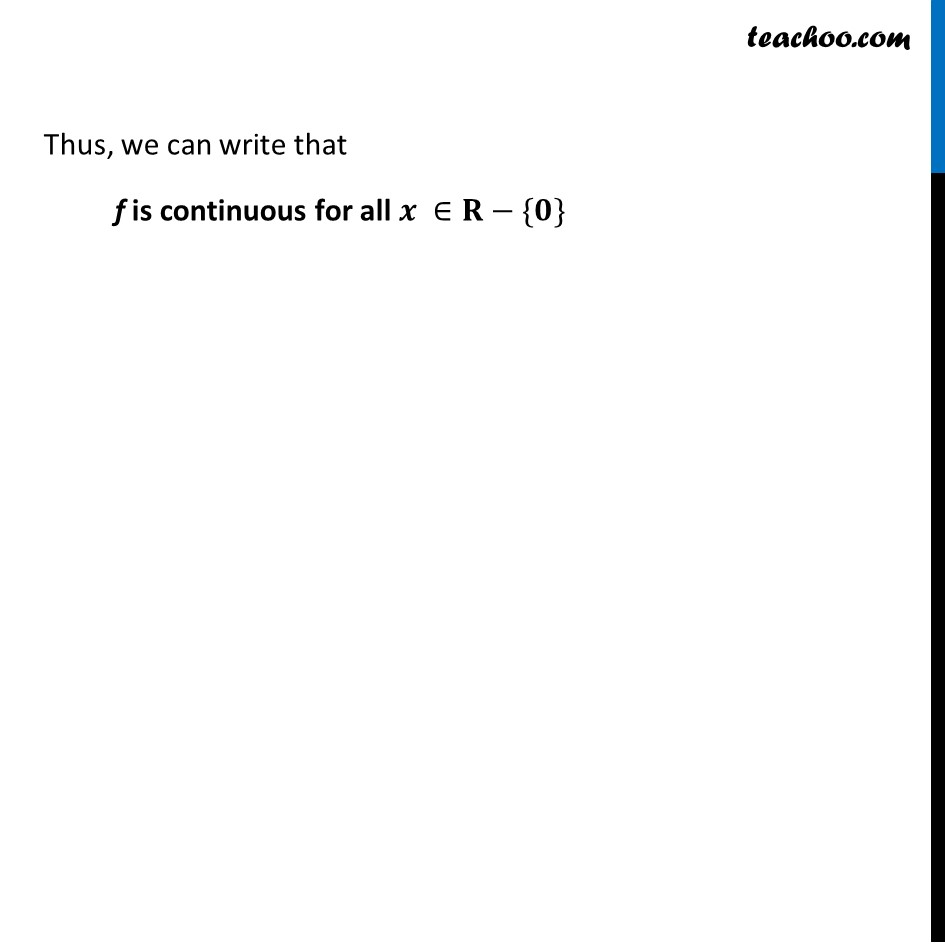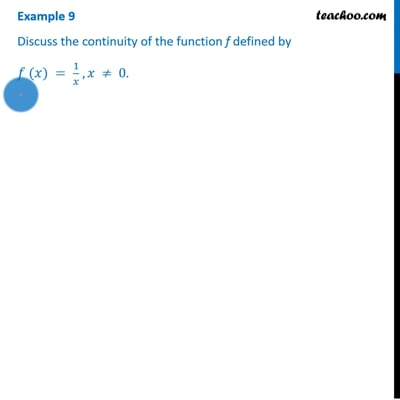Checking continuity at any point

Chapter 5 Class 12 Continuity and Differentiability
Concept wiseThis video is only available for Teachoo black users

Introducing your new favourite teacher - Teachoo Black, at only ₹83 per month

### Transcript

Example 9 Discuss the continuity of the function f defined by 𝑓 (𝑥) = 1/𝑥 , 𝑥 ≠ 0. Given 𝑓 (𝑥) = 1/𝑥 At 𝒙 = 𝟎 𝑓 (0) = 1/0 = ∞ Hence, 𝑓(𝑥) is not defined at 𝒙=𝟎 By definition, 𝑓 (𝑥) = 1/𝑥 , 𝑥 ≠ 0. So, we check for continuity at all points except 0. Let c be any real number except 0. f is continuous at 𝑥 =𝑐 if , (𝐥𝐢𝐦)┬(𝐱→𝒄) 𝒇(𝒙)=𝒇(𝒄) Since, L.H.S = R.H.S ∴ Function is continuous at x = c (Except 0) (𝐥𝐢𝐦)┬(𝐱→𝒄) 𝒇(𝒙) = lim┬(x→𝑐) 1/𝑥 Putting 𝑥 =𝑐 = 1/𝑐 𝒇(𝒄) =1/𝑐 " " Thus, we can write that f is continuous for all 𝒙 ∈𝐑−{𝟎} Thus, we can write that f is continuous for all 𝒙 ∈𝐑−{𝟎}